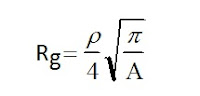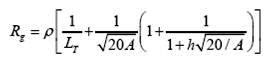### Ground Resistance Calculations

In Article " Introduction to Earthing System ", I explained the following points:

1. Introduction
2. Determining Ground Resistance
3. Soil Resistivity

Today, I will explain how to calculate the Ground Resistance.

2.2 Calculating Ground Resistance

The following formula (source: IEEE Std.142:1991) enable the resistance to ground to be calculated.Where:

R = resistance in Ω
ρ =  resistivity in Ω.cm
d = distances - in cm

S =  space between ground rods

Multiple Ground Rods space factor will be as follows:

2.2.1 Calculating Ground Resistance for substations

Ideally, a ground system should be as close to zero resistance as possible. For most transmission and other larger substations, the ground resistance should be about 1Ω or less. In smaller distribution substations the usually acceptable range is from 1-5Ω, depending on local conditions. Estimation of the total resistance to remote ground is one of the first steps in determining the size and basic layout of a grounding system.

A minimum value of the substation grounding resistance in uniform soil can be estimated by means of the formula of a circular metal plate at zero depth once the soil resistivity has been determined.

Use the following formula to estimate the minimum resistance that can be expected in designing a grounding system:Where:

Rg = ground resistance in Ω.

ρ = average earth resistivity in Ω/m.

A = area occupied by the ground grid in square meters.

Π = 3.14

Example#1:

What is the grid resistance of a system if
ρ  = 250 Ω/m and A = 3500 m2?

Solution:

Calculating with the above formula the results are as follows:So, Rg = 1.87 Ω

Next, an upper limit of the substation resistivity can be obtained by adding a second term to the above formula. The second term recognizes the fact that the resistance of any actual grounding system that consists of a number of conductors is higher than that of a solid metallic plate. This difference will decrease with the increasing length of buried conductors, approaching 0 for infinite L, when the condition of a solid plate is reached. (IEEE-80)

To estimate the upper limit, use the formula:Where:

Rg = ground resistance in Ω.

ρ = average earth resistivity in Ω/m. This measurement should be located on the prints or the 1000 Ω/m can be used.

A = area occupied by the ground grid in square meters.

L = total buried length of conductors in meters.

Π = 3.14

 Note#1Use the above formula to approximate the ground resistance of a system and not as a replacement for actual ground measurements.

The total burial length is the combination of the horizontal and vertical conductors in the grid as well as the ground rods. L can be calculated as:Where:

LC = total length of grid conductor (m)

LR = total length of ground rods (m)

A better approximation was determined to include the grid depthWhere

h : depth of the grid (m)

This equations shows that a larger the area and the greater the total length of the grounding conductor used would resulting a lower ground grid resistance.

3- Verifying Ground Grid Conductor Installation

Your verification of a grid system starts with inspections of the station layout plan, showing all major equipment and structures.

The area of the grounding system is the single most important geometrical factor in determining the resistances of the grid. Larger grounded areas result in lower grid resistance and thus, lower GPR and mesh voltages.

The design of the ground grid is based on three main parameters:

1. Maximum prospective ground fault current passing between grounding system and the body of earth,
2. Duration of flow of this current (based on a duration of 1 second),
3. Soil resistivity and the nature of the ground at the site.

 Note#2It is not possible to use the short-time current rating of the breakers, or three seconds, for the first two of the above parameters. Even in areas of low soil resistivity it would be difficult, if not impossible, to design an electrode adequate for such a duty. It is therefore necessary to determine the maximum current, and its duration of flow (1 second given by design), which the electrode must safely transmit to or from the body of earth.

3.1 Design Guidelines and Requirements

• A continuous conductor loop surrounds the perimeter to enclose as much area as practical. This practice helps to avoid high current concentration and hence high gradients both in the grid area and near the projecting cable ends. Enclosing more area also reduces the resistance of the grounding grid.
• Within the loop, the conductors lay in parallel lines and, where practical, along the structures or rows of equipment, to provide for short ground connections.
• A typical grid system for a substation may include 70 or 120 square millimeters (mm2), No. 4/0 or 2/0 AWG bare copper conductors buried 18 inches (0.5 m) below grade, minimum, spaced 10 to 20 feet (3 to 6 m) apart, in a grid pattern. At cross-connections, securely bond the conductors together by thermite welding, brazing, or approved compression connectors. Ground rods should be placed at the grid corners and never less than 6 feet apart by design.
• A grid system normally extends over the entire substation switchyard and often beyond the fence line. Some codes require a grounding conductor to be buried approximately 3 feet (1m) outside and parallel to the fence. Use multiple ground leads or larger sized conductors where high concentrations of current may occur, such as a neutral-to-ground connection of generators, capacitor banks, or transformers.
• The ratio of the sides of the mesh is usually from 1:1 to 1:3, unless a precise analysis warrants more extreme values. Frequent cross-connections have a relatively small effect on lowering the resistance of a grid, but are useful in securing multiple paths for fault current conditions.
• Wire size of 35 mm2 (2 AWG) or larger must be stranded.
• Some codes require that tinned wires shall be used where the resistivity of the soil is less than 70 Ω/m.
• Sharp bends must be avoided in all grounding conductors. (This refers to above ground connections.)

In the next Article, I will explain Ground Resistance Measurement. Please, keep following.